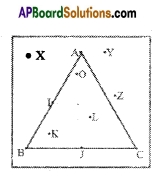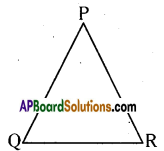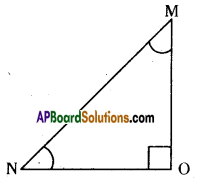AP State Syllabus AP Board 6th Class Maths Solutions Chapter 9 2D-3D Shapes Ex 9.2 Textbook Questions and Answers.

## AP State Syllabus 6th Class Maths Solutions 9th Lesson 2D-3D Shapes Ex 9.2Question 1.
Look at the given triangle and answer the following questions.
i) Which points are marked in the exterior of the triangle?
ii) Which points are marked on the triangle?
iii) Which points are marked in the interior of the triangle?Solution:
i) The points marked in the exterior of the triangle are X, Y and Z.
ii) The points marked on the triangle are A, I, B, J and C.
iii) The points marked in the interior of the triangle are K, L and O.

Question 2.
i) How many sides are there in the triangle? What are they?
ii) How many vertices lie there on the triangle? What are they?
iii) What is the side opposite to the vertex P?
iv) What is the vertex opposite to $$\overline{\mathrm{PR}}$$ ?Solution:
In the given ∆PQR,
i) It has three sides. They are $$\overline{\mathrm{PQ}}, \overline{\mathrm{QR}}$$ and $$\overline{\mathrm{PR}}$$
ii) It has three vertices. They are P, Q and R.
iii) The side opposite to the vertex P is $$\overline{\mathrm{QR}} iv) The vertex opposite to the side [latex]\overline{\mathrm{PR}}$$ is Q.Question 3.
Look at the given triangle and answer the following questions.
i) How many angles are there in the triangle? What are they?
ii) What is the angle opposite to $$\overline{\mathrm{MN}}$$ ?
iii) Where is the right angle in the given triangle ?Solution:
In the given ∆MON,
i) It has three angles, they are ∠MNO, ∠NOM and ∠OMN.
ii) Angle opposite to the side $$\overline{\mathrm{MN}}$$ is ∠MON or ∠O.
iii) In the triangle ∠MON is the right angle.